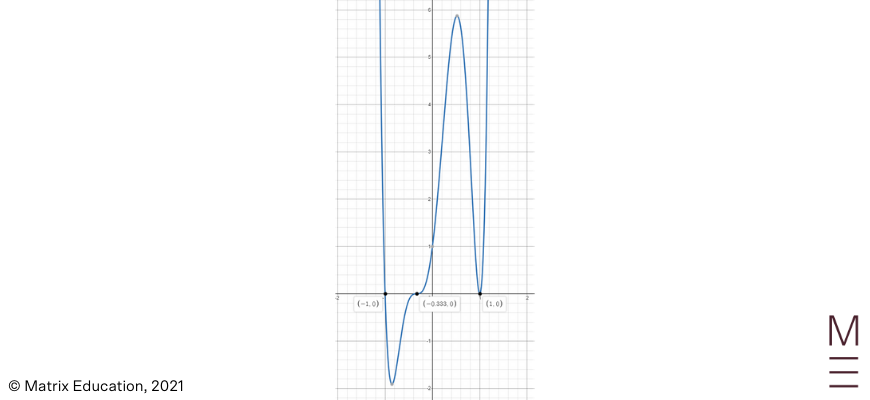# Part 1: Polynomials | Further Functions and Relations

Stuck on polynomials? Well, in this article, we'll explain what they are, how to solve them, and provide you with some examples to work through so your marks only have a positive function.In this article, we will be utilising our knowledge of finding $$x$$ – intercepts, $$y$$ – intercepts and sketching various non-linear curves to sketch complicated Polynomial curves.

As you already know, a Polynomial is an algebraic expression made up of multiple terms. In this article, we will break down some complex Polynomial curves in a clear and accessible manner. We will then help you apply your knowledge with some concept check questions of varying difficulty at the end of the article.

## Year 11 Advanced Mathematics: Polynomials

Mastering Polynomial sketching plays an important role in being able to visualise certain graphs which will come up in later topics. It is one important part of understanding the nature behind various graphs and the effect different parts of an equation have on a final curve.

We’re going to look at the following NESA syllabus points.

## NESA Syllabus Outcomes

NESA dictates that students need to learn the following concepts to demonstrate proficiency in Polynomials.

### F1.4 Further functions and relations

• Define a real polynomial $$P(x)$$ as the expression
 $$a_n x^n+a_{n-1} x^{n-1}+⋯+a_2 x^2+a_1 x+a_0$$

where $$n=0,1,2,…$$ and $$a_0,a_1,a_2,…,a_n$$ are real numbers

• Identify the coefficients and the degree of the polynomial (ACMMM015)
• Identify the shape and features of graphs of polynomial functions of any degree in factored form and sketch their graphs

## Assumed Knowledge

Students should already be familiar with function notation, domain and range, odd/even functions and graphing non-linear functions.

This content can be found in our Year 10 Guides should students want to refresh their understanding:

To begin with, let’s get a clearer understanding of what a Polynomial is.

## Further Functions and Relations – Polynomials

### Polynomials

A polynomial is an algebraic expression made up of many terms as seen in the equation below.

 $$P(x)=a_n x^n+a_{n-1} x^{n-1}+a_{n-2}x^{n-2}⋯+a_2 x^2+a_1 x+a_0$$

It has four terms which need to be familiarized with as this determines what the polynomial graphs look like when we learn how to sketch it later. Let’s take a look at this equation and its individual parts and see how they work:

 Polynomial Explanation $$a_n x^n$$ The leading term is $$a_n x^n$$ and is the term with the highest power. $$n$$ The degree is the highest power in the polynomial expression. $$a_n$$ $$a_n$$ is the leading coefficient as it is connected to the term with the highest power. The other values $$a_{n-1},a_{n-2},a_3,a_2,a_1$$ are all coefficients. $$a_0$$ $$a_0$$ is the constant term.

### Example polynomials

Below are some Polynomials you are already familiar with:

• $$y=x-1$$

This is a linear expression which is also a polynomial of degree 1.

• $$y=x^2+7x+6$$

This is a quadratic expression which is also a polynomial of degree 2.

• $$y=x^3-1$$

This is a cubic expression which is also a polynomial of degree 3.

• $$y=x^4+x^3+x^2+x+1$$

This is a quartic expression which is also a polynomial of degree 4.

## Want to get more practice with polynomials?

With Matrix+, we provide you with clear and structured online lesson videos, quality resources, and forums to ask your Matrix teachers questions and for feedback.## Identifying the roots of the polynomial

When sketching a polynomial, it’s important to find the $$x$$-intercepts and identify the nature of the roots as either single, double or triple roots.

### Example

Determine the nature of the roots for the following polynomial:

$$P(x)=(2x-5) (x+1)^2 (x-1)^3$$

When we calculate the $$x$$-intercepts for each polynomial, we let $$y=0$$ leaving us with the equation:

$$0=(2x-5) (x+1)^2 (x-1)^3$$

This leaves us with three equations to solve to find the $$x$$-intercepts:

\begin{align*}
(2x-5)=0 \\
(x+1)^2=0 \\
(x-1)^3=0
\end{align*}

### Single root

$$x=\frac{5}{2}$$. This is a single root because the power of the brackets is 1. As a single root, the curve will pass through the $$x$$-axis as follows:It’s important to note that the power of the brackets will impact the nature of the roots. Solving each equation, we get the following $$x$$ -intercepts: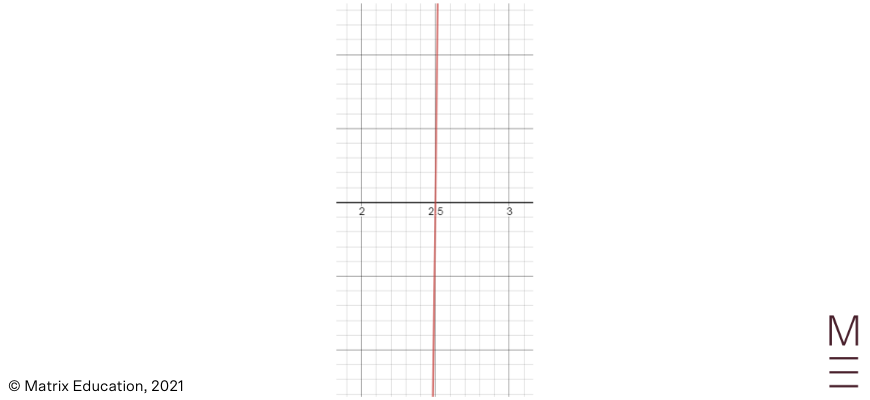### Double root

$$x=-1$$. This is a double root because the power of the brackets is 2. As a double root, the curve will pass through the $$x$$-axis as follows: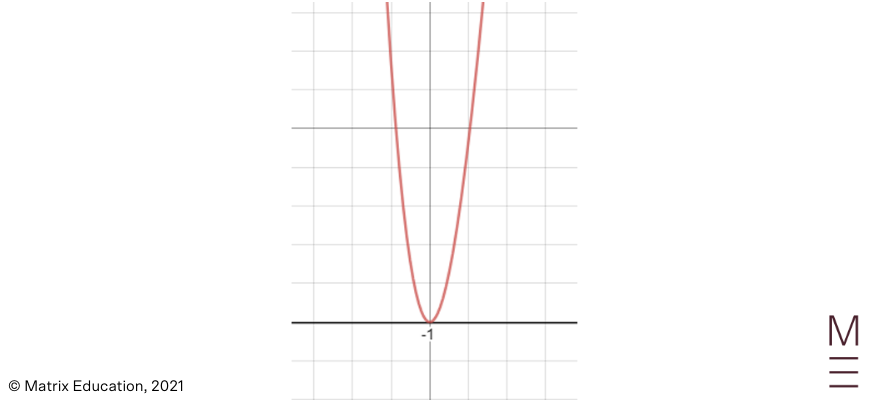### Triple root

$$x=1$$. This is a triple root because the power of the brackets is 3. As a triple root, the curve will pass through the $$x$$-axis as follows: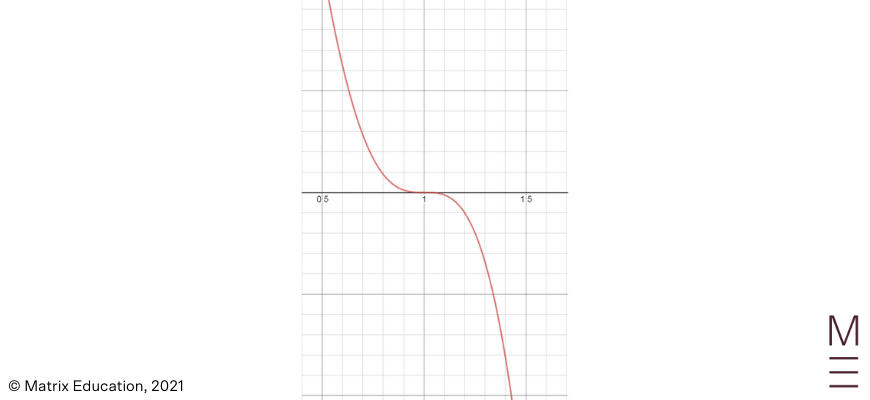## General shape of the polynomial curve

### Positive odd degree polynomials

If the polynomial curve has an odd degree and is positive, it will result in the curve beginning in the 3rd quadrant and ending in the 1st quadrant as follows: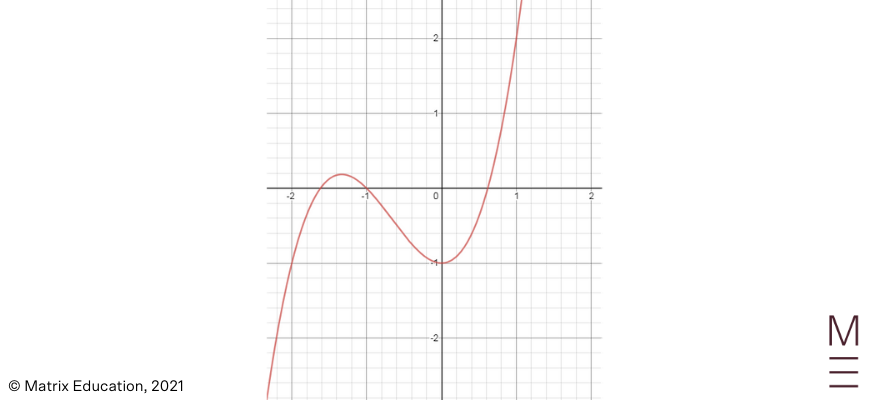### Negative odd degree polynomials

If the polynomial curve has an odd degree and is negative, it will result in the curve beginning in the 2nd quadrant and ending in the 3rd quadrant as follows: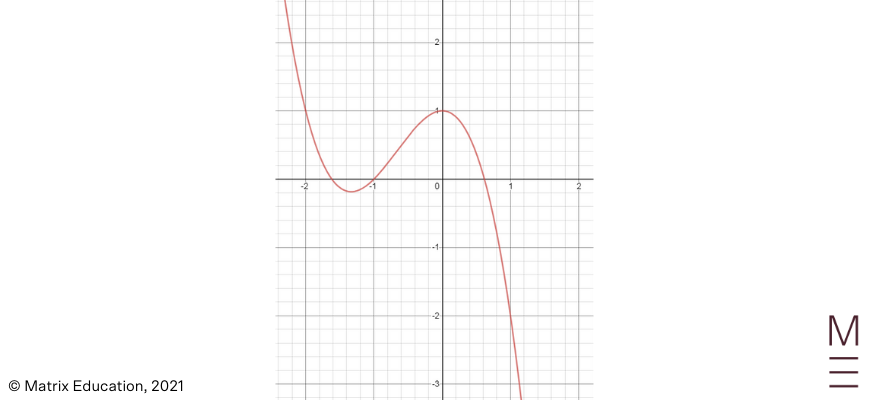### Positive even degree polynomials

If the polynomial has an even degree and is positive, it will result in the curve beginning and ending in the 1st and 2nd quadrants as follows: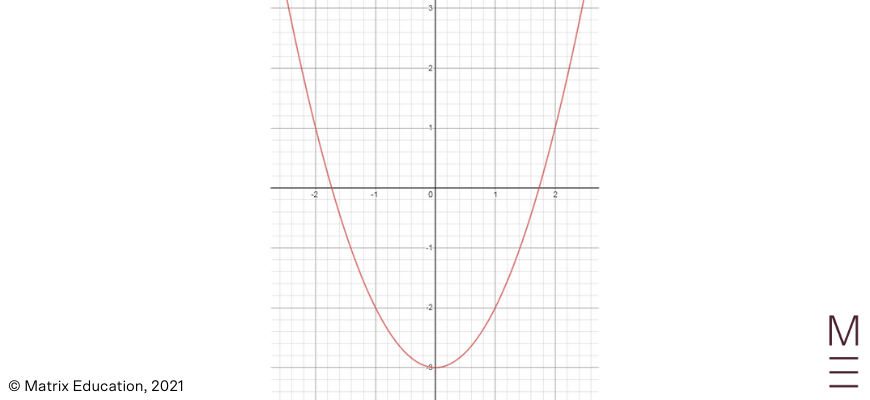### Negative even degree polynomials

If the polynomial has an even degree and is negative, it will result in the curve beginning and ending in the 3rd and 4th quadrants as follows: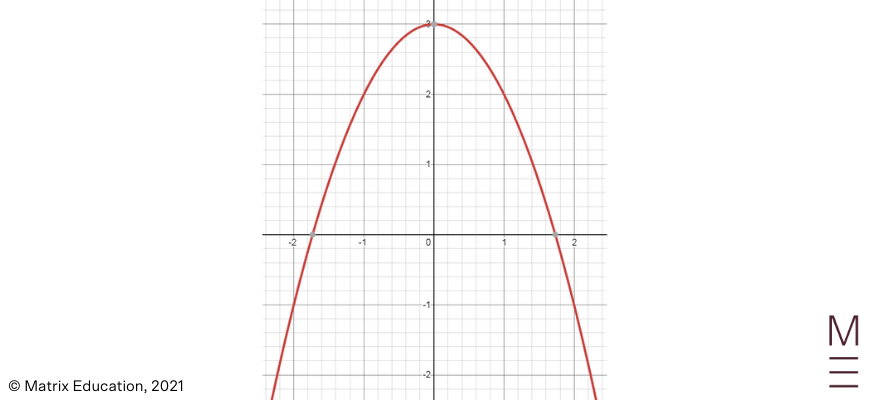## Sketching polynomials with varying roots

Consider the following process that we have broken down into 5 steps for sketching polynomials:

 Steps Explanation Step 1 The degree of the polynomial. This will determine the general shape of the curve. Step 2 The $$x$$ – intercepts of the curve. Step 3 The $$y$$ – intercepts of the curve. Step 4 Determine the type of root for each $$x$$ – intercept. This will reveal to you if the curve:Passes through the axis (single root)Bounces off the axis (double root), orPasses through and is horizontal at the axis (triple root). Step 5 Combine all this information and sketch it on the Cartesian plane.

Now let’s put this into practice.

### Example 1:

Sketch the following polynomial, clearly indicating all intercepts on the coordinate axes:

$$P(x)=(x-1)(x+1)(x+3)$$

### Solution 1:

Step 1: Multiplying all the values together, the highest power of $$x$$ will be $$3$$. Therefore, this polynomial has a degree of $$3$$ and will extend from the 3rd quadrant to the 1st quadrant.

Step 2: When finding the $$x$$-intercepts, let $$y=0$$.

$$0=(x-1)(x+1)(x+3) \\ x=-3,-1,1$$

Step 3: When finding the $$y$$ – intercepts, let $$x=0$$.

$$P(0)=(0-1)(0+1)(0+3)=-3$$

Step 4: All of the $$x$$-intercepts have a single root.

Step 5: Therefore, placing all this information together we obtain the following graph: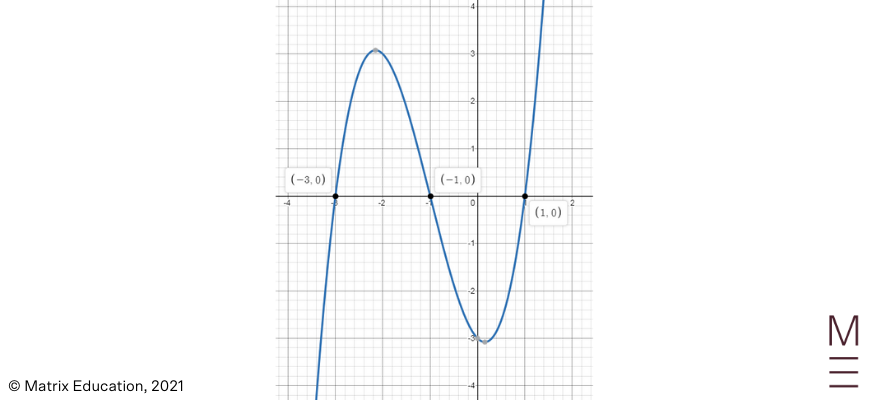### Example 2:

Sketch the following polynomial, clearly indicating all intercepts on the coordinate axes:

$$P(x)=(1-x) (x-3)^2 (x+1)$$

### Solution 2:

Step 1: Multiplying all the values together, the highest power of $$x$$ will be $$4$$, and the coefficient will also be $$“-“$$.
Therefore, this negative polynomial has a degree of $$4$$ and will extend from the 1st quadrant to the 4th quadrant.

Step 2: When finding the $$x$$ – intercepts, let $$y=0$$

$$0=(1-x) (x-3)^2 (x+1) \\ x=-1,1,3$$

Step 3: When finding the y-intercepts, let $$x=0$$

$$P(0)=(1-0) (0-3)^2 (0+1)=9$$

Step 4: The $$x$$-intercepts $$x=-1$$ and $$1$$ are singular roots, and the $$x$$-intercept $$x=3$$ is a double root.

Step 5: Therefore, placing all this information together we obtain the following graph: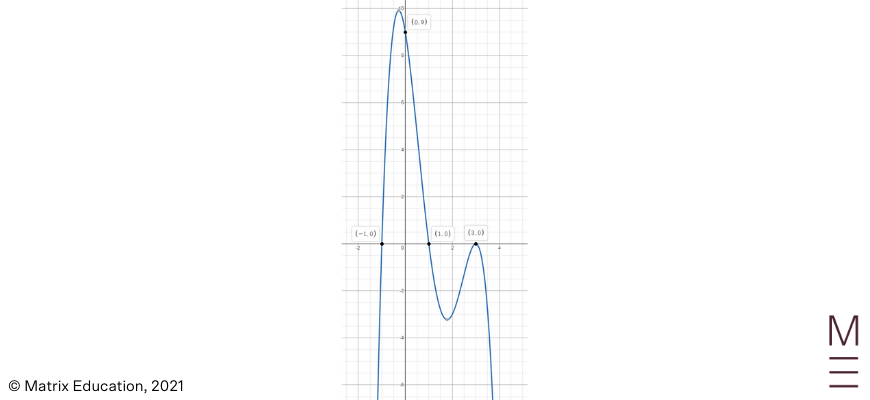### Example 3:

Sketch the following polynomial, clearly indicating all the intercepts on the coordinate axes:

$$P(x)=x(x-2)^3 (x+1)^2$$

### Solution 3:

Step 1: Multiplying all the values together, the highest power of $$x$$ will be $$6$$. Therefore, this polynomial has a degree of $$6$$ and will extend from the 1st quadrant to the 3rd quadrant.

Step 2: When finding the $$x$$-intercepts, let $$y=0$$

$$0=x(x-2)^3 (x+1)^2 \\ x=-1,0,2$$

Step 3: When finding the $$y$$-intercepts, let $$x=0$$

$$P(0)=0(0-2)^3 (0+1)^2=0$$

Step 4: The $$x$$-intercept $$x=0$$ is a singular root, $$x=2$$ is a triple root, and $$x=-1$$ is a double root.

Step 5: Therefore, placing all this information together we obtain the following graph: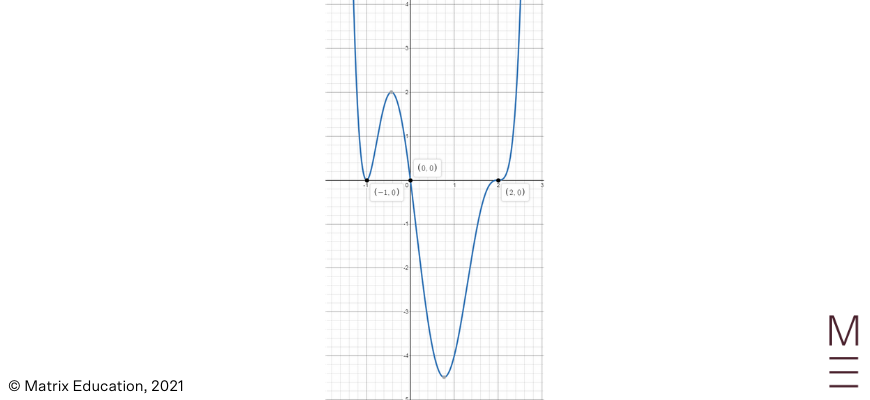## Concept Check Questions

1. Sketch the following polynomial: $$y=(2x+1)^2(x-1)^2$$ labelling all $$x$$ and $$y$$ intercepts.

2. Sketch the following polynomial: $$y=x^2(2x-3)^3$$ labelling all $$x$$ and $$y$$ intercepts.

3. Sketch the following polynomial: $$y=(3x+1)^3(x+1)(x-1)^2$$ labelling all $$x$$ and $$y$$ intercepts.

1.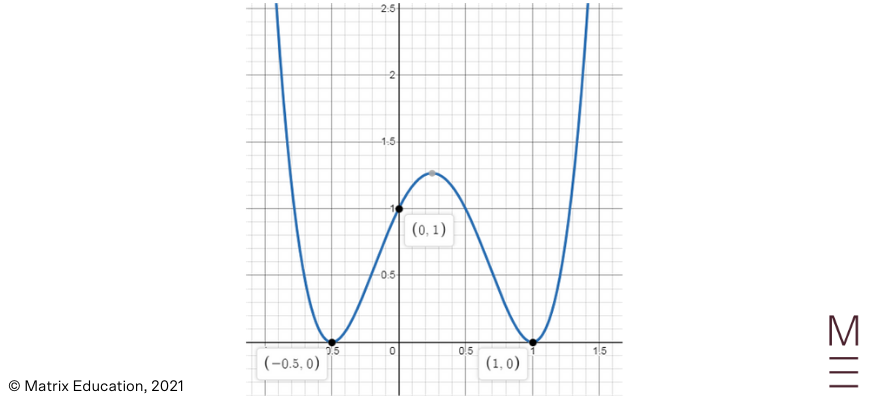2.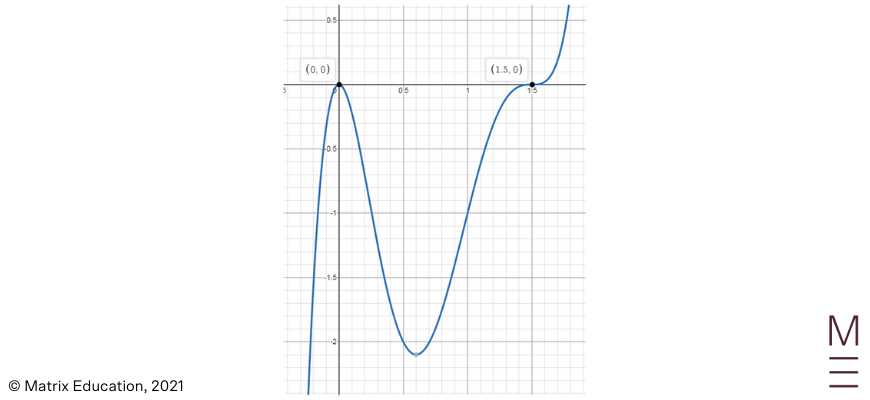3.